Normal view

# Models for discrete longitudinal data / Geert Molenberghs, Geert Verbeke.

Material type:TextLanguage: English Series: Springer series in statistics: Publisher: New York ; London : Springer, c2006Description: xxii, 683 p. : ill. 25 cmISBN: 0387251448 (hbk.); 9780387251448DDC classification: 519.53 LOC classification: QA278 | .M65 2005Online resources: Publisher description | WorldCat details | Ebook Fulltext
Contents:
Table of contents Introduction -- Motivating studies -- Generalized linear models -- Linear mixed models for Gaussian longitudinal data -- Model families -- The strength of marginal models -- Likelihood-based marginal models -- Generalized estimating equations -- Pseudo-likelihood -- Fitting marginal models with SAS -- Conditional models -- Pseudo-likehood -- From subject-specific to random-effects models -- The generalized linear mixed model (GLMM) -- Fitting generalized linear mixed models with SAS -- Marginal versus random-effects models The analgesic trial -- Ordinal data -- The epilepsy data -- Non-linear models -- Pseudo-likelihood for a hierarchical model -- Random-effects models with serial correlation -- Non-Gaussian random effects -- Joint continuous and discrete responses -- High-dimensional joint models -- Missing data concepts -- Simple methods, direct likelihood, and WGEE -- Multiple imputation and the EM algorithm -- Selection models -- Pattern-mixture models -- Sensitivity analysis -- Incomplete data and SAS.
Summary: Summary: The linear mixed model is the main parametric tool for the analysis of continuous longitudinal data. This book shows how the different approaches can be implemented within the SAS software package.
Tags from this library: No tags from this library for this title.Average rating: 0.0 (0 votes)
Item type Current location Collection Call number Copy number Status Date due Barcode Item holdsE-Book
E-book
Non-fiction 519.53 MOM 2006 (Browse shelf) Not for loanText
Reserve Section
Non-fiction 519.53 MOM 2006 (Browse shelf) C-1 Not For Loan 26628Text
Circulation Section
Non-fiction 519.53 MOM 2006 (Browse shelf) C-2 Available 26884Text
Circulation Section
Non-fiction 519.53 MOM 2006 (Browse shelf) C-3 Available 26885
Total holds: 0
##### Browsing EWU Library shelves, Shelving location: E-book Close shelf browser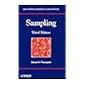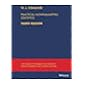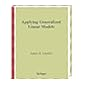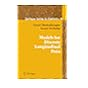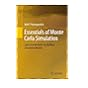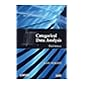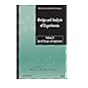519.52 THS 2012 Sampling / 519.53 COP 2013 Practical nonparametric statistics / 519.53 LIA 1997 Applying generalized linear models / 519.53 MOM 2006 Models for discrete longitudinal data / 519.53 THE 2013 Essentials of Monte Carlo simulation : 519.535 AGC 2013 Categorical data analysis / 519.535 DES 2012 Design and analysis of experiments.

Includes bibliographical references and index.

Table of contents Introduction --
Motivating studies --
Generalized linear models --
Linear mixed models for Gaussian longitudinal data --
Model families --
The strength of marginal models --
Likelihood-based marginal models --
Generalized estimating equations --
Pseudo-likelihood --
Fitting marginal models with SAS --
Conditional models --
Pseudo-likehood --
From subject-specific to random-effects models --
The generalized linear mixed model (GLMM) --
Fitting generalized linear mixed models with SAS --
Marginal versus random-effects models The analgesic trial --
Ordinal data --
The epilepsy data --
Non-linear models --
Pseudo-likelihood for a hierarchical model --
Random-effects models with serial correlation --
Non-Gaussian random effects --
Joint continuous and discrete responses --
High-dimensional joint models --
Missing data concepts --
Simple methods, direct likelihood, and WGEE --
Multiple imputation and the EM algorithm --
Selection models --
Pattern-mixture models --
Sensitivity analysis --
Incomplete data and SAS.

Summary:
The linear mixed model is the main parametric tool for the analysis of continuous longitudinal data. This book shows how the different approaches can be implemented within the SAS software package.

Applied Statistics

There are no comments for this item.

to post a comment.
Share

Library Home | Contacts | E-journals
Copyright @ 2011-2019 EWU Library
East West University